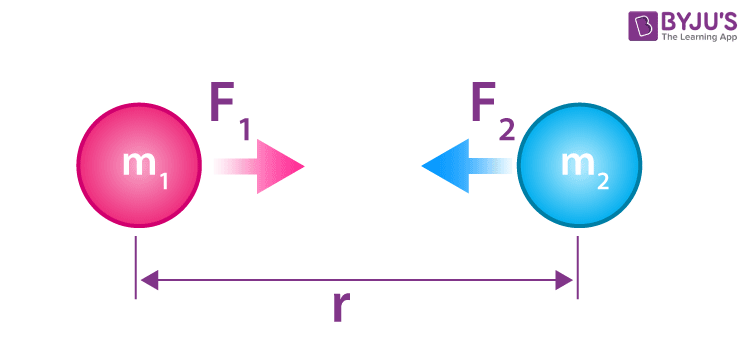What is Newton's law of universal gravitation in simple terms?

Newton’s Law of Universal Gravitation states that every particle attracts every other particle in the universe with force directly proportional to the product of the masses and inversely proportional to the square of the distance between them.

Definition

Newton’s Law of Universal Gravitation states that every particle attracts every other particle in the universe with force directly proportional to the product of the masses and inversely proportional to the square of the distance between them.

Universal Gravitation Equation$$F\propto \frac{m_{1}m_{2}}{r^2}$$ $$F= G\frac{m_{1}m_{2}}{r^2}$$

Where,

• F is the gravitational force between bodies
• m1 and m2 are the masses of the bodies
• r is the distance between the centres of two bodies
• G is the universal gravitational constant (6.673 x 10-11 N m2/kg2)• In 1798, the Cavendish experiment, conducted by British scientist Henry Cavendish, was the first laboratory test of Newton’s theory of gravitation between masses.
• Newton’s law of gravitation resembles Coulomb’s law of electrical forces, which is used to measure the magnitude of the electrical force produced between two charged bodies. Both are inverse-square laws, where force is inversely proportional to the square of the distance between the bodies.

Articles to Explore:(2)(0)